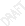# High School Mathematics Electives Required to Be Offered

 Date 26.04.2018 Size 43.95 Kb.High School Mathematics Electives Required to Be Offered Optional Electives Transition year 2012-2013 Algebra I or Math I Algebra II Algebra III Geometry or Applied Geometry Pre-Calculus Trigonometry Conceptual Mathematics Transition Mathematics for Seniors Calculus Probability and Statistics Mathematics College Courses AP Mathematics Courses Algebra Support or Math I Lab Transition year 2013-2014 Algebra I or Math I Algebra II Algebra III Geometry or Applied Geometry or Math II Pre-Calculus Trigonometry Conceptual Mathematics Transition Mathematics for Seniors Calculus Probability and Statistics Mathematics College Courses AP Mathematics Courses Algebra Support or Math I Lab 2014-2015 (Required new course – Math I) Math I Geometry or Math II Algebra II or Math III LA , Math III STEM, Math III TR Algebra III Pre-Calculus Trigonometry Conceptual Mathematics Transition Mathematics for Seniors Math I Lab Math IV AP Calculus Advanced Mathematical Modeling STEM Readiness Mathematics AP Computer Science Calculus Math IV Technical Readiness Other College Level Mathematics Courses 2015-2016 (Required new course – Math II)

Math I Math I Lab Math II Math IV Algebra II or Math III LA , Math III STEM, AP Calculus

Math III TR Calculus

Pre-Calculus AP Statistics

Conceptual Mathematics AP Computer Science

Transition Mathematics for Seniors Math IV Technical Readiness

Other College Level Mathematics Courses

 2016-2017 (Required new courses – Math III LA, Math III STEM, Math III Technical Readiness)

Math I Math I Lab Math II Math IV Math III LA AP Calculus

Math III STEM Advanced Mathematical Modeling

Pre-Calculus AP Statistics

Transition Mathematics for Seniors STEM Readiness Mathematics

AP Computer Science

Calculus

Other College Level Mathematics Courses

 2017-2018 (Required new courses - Math IV Technical Readiness, Math IV)

Math I Math I Lab Math II AP Calculus Math III LA Advanced Mathematical Modeling

Math III STEM AP Statistics

Math IV AP Computer Science

Transition Math for Seniors Other College Level Courses

4th Course Descriptions

High School Math IV

The fundamental purpose of Mathematics IV is to generalize and abstract learning accumulated through previous courses and to provide the final springboard to calculus. Students take an extensive look at the relationships among complex numbers, vectors, and matrices. They build on their understanding of functions, analyze rational functions using an intuitive approach to limits and synthesize functions by considering compositions and inverses. Students expand their work with trigonometric functions and their inverses and complete the study of the conic sections begun in Mathematics II. They enhance their understanding of probability by considering probability distributions. Previous experiences with series are augmented.  High School Math IV is appropriate for those students that complete Math III STEM.

This course is designed for students who have completed the Math III (LA) course and subsequently decided they are interested in pursuing a STEM career. It includes standards that would have been covered in Math III (STEM) but not in Math III (LA) (i.e. standards in the CCSS document that are marked with a “+” ), selected topics from the suggested CCSS Math IV course, and topics drawing from standards covered in Math I and Math II as needed for coherence.

Students continue to build upon their algebra and geometry foundations and expand their understanding through further mathematical experiences. The primary focal points of Advanced Mathematical Modeling include the analysis of information using statistical methods and probability, modeling change and mathematical relationships, mathematical decision making in finance, and spatial and geometric modeling for decision-making. Students learn to become critical consumers of the quantitative data that surround them every day, knowledgeable decision makers who use logical reasoning and mathematical thinkers who can use their quantitative skills to solve problems related to a wide range of situations. As they solve problems in various applied situations, they develop critical skills for success in college and careers, including investigation, research, collaboration and both written and oral communication of their work. As students work with these topics, they continually rely on mathematical processes, including problem-solving techniques, appropriate mathematical language and communication skills, connections within and outside mathematics and reasoning. Students also use multiple representations, technology, applications and modeling and numerical fluency in problem-solving contexts.

Transition Mathematics for Seniors*

Transition Math for Seniors prepares students for their entry-level credit-bearing liberal studies mathematics course at the post-secondary level. This course will solidify their quantitative literacy by enhancing numeracy and problem solving skills as they investigate and use the fundamental concepts of algebra, geometry, and introductory trigonometry.  * See current policy for placement criteria.

Other Available Courses that have not been described here:  AP Calculus, AP Computer Science, AP Statistics, Calculus, Math I Lab (Must be taken during 9th grade year), Technical Readiness Mathematics III & IV, Other College Level Mathematics courses.

West Virginia Mathematics

Possible Course Sequences during transition
Recommended course sequences listed here are not inclusive. These are not complete lists of every possible pathway to graduation.

Other 4th course options:

AP Computer Science

Transition Math for Seniors

STEM Readiness Other College Level course

West Virginia Mathematics

Possible Course Sequences upon complete transition

Recommended course sequences listed here are not inclusive. These are not complete lists of every possible pathway to graduation.

Other 4th course options:

Please note that Policies 2510 and 2520.2b are being placed on public comment soon – with State Board approval HS Math course names change to:

Math I to Math 9

Math II to Math 10

Math III to Math 11

Math IV to Math 12

AP Computer Science

Transition Math for Seniors

Other College Level courses